maths > mensuration-high

Pyramids : Surface Area and Volume

what you'll learn...

Overview

Surface Area and Volume of pyramid: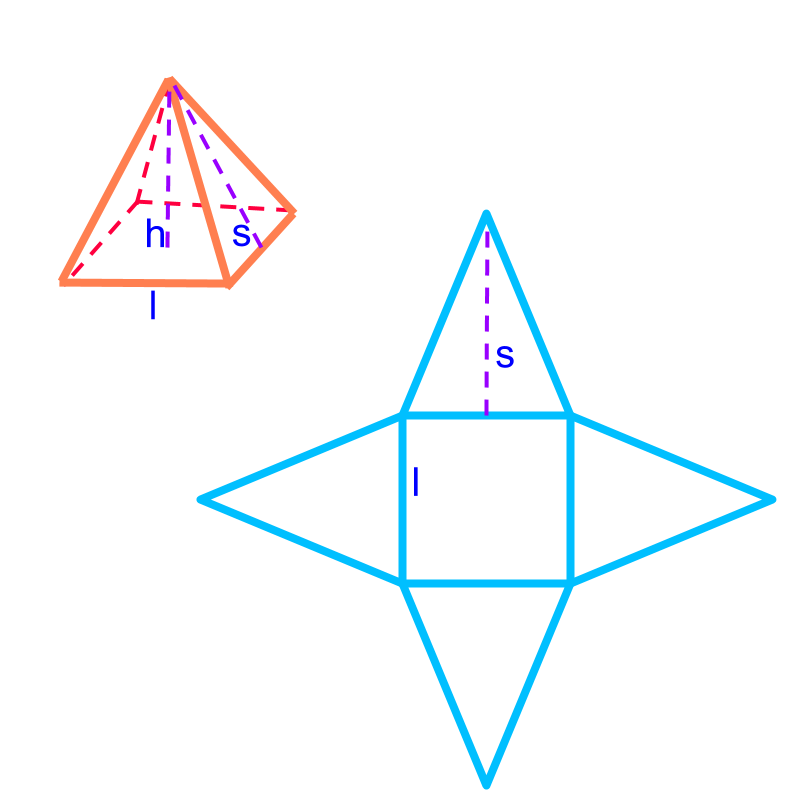Total surface area of the pyramid
$=$$=$ area of the base-surface$+$$+$ sum of the area of the side-faces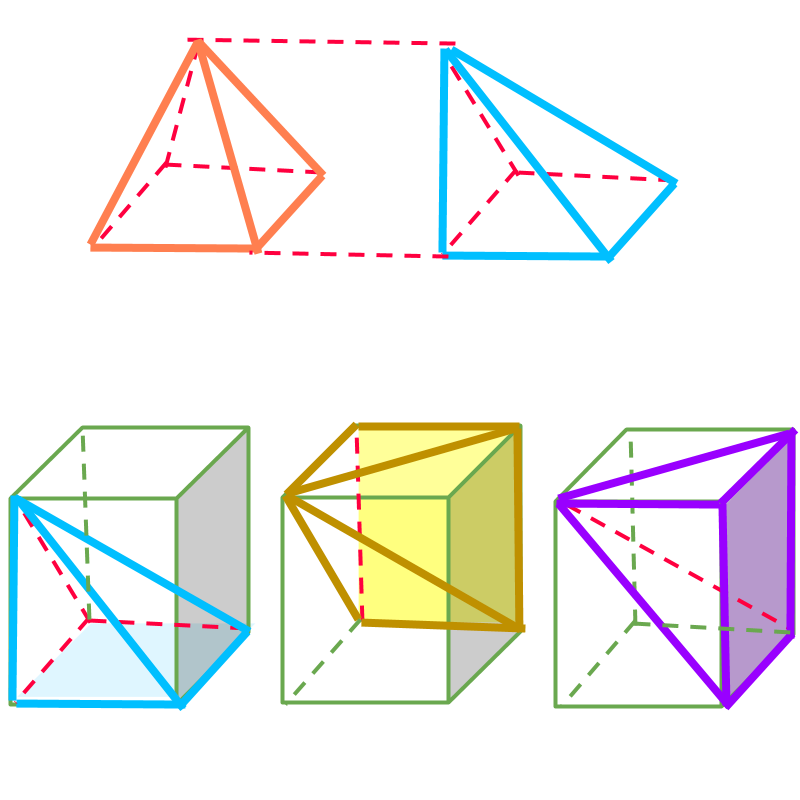Volume of the pyramid
$=\frac{1}{3}×\phantom{\rule{1ex}{0ex}}\text{area of the base-face}\phantom{\rule{1ex}{0ex}}×\phantom{\rule{1ex}{0ex}}\text{height}$$= \frac{1}{3} \times \textrm{a r e a o f t h e b a s e - f a c e} \times \textrm{h e i g h t}$

pyramid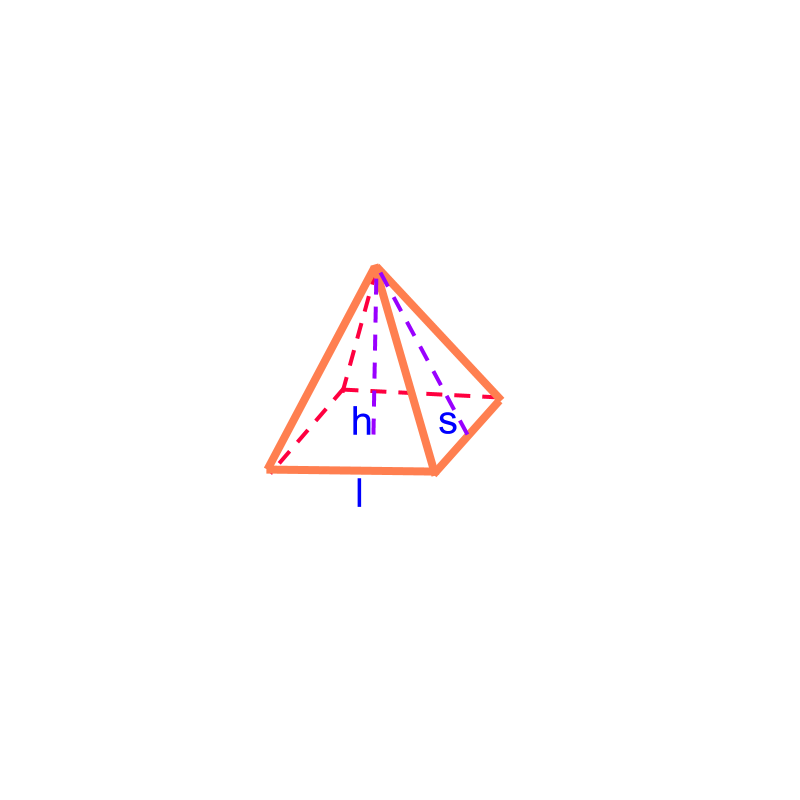The shape shown in the figure is "a pyramid". Pyramid is a 3D solid shape with a 2D-polygon base at the bottom with triangular faces on the sides converging to a single point on the top . The base can be any 2D-polygon, for example, square, rectangle, triangle, etc, and the pyramids are square-pyramid, rectangular-pyramid, triangular-pyramid respectively.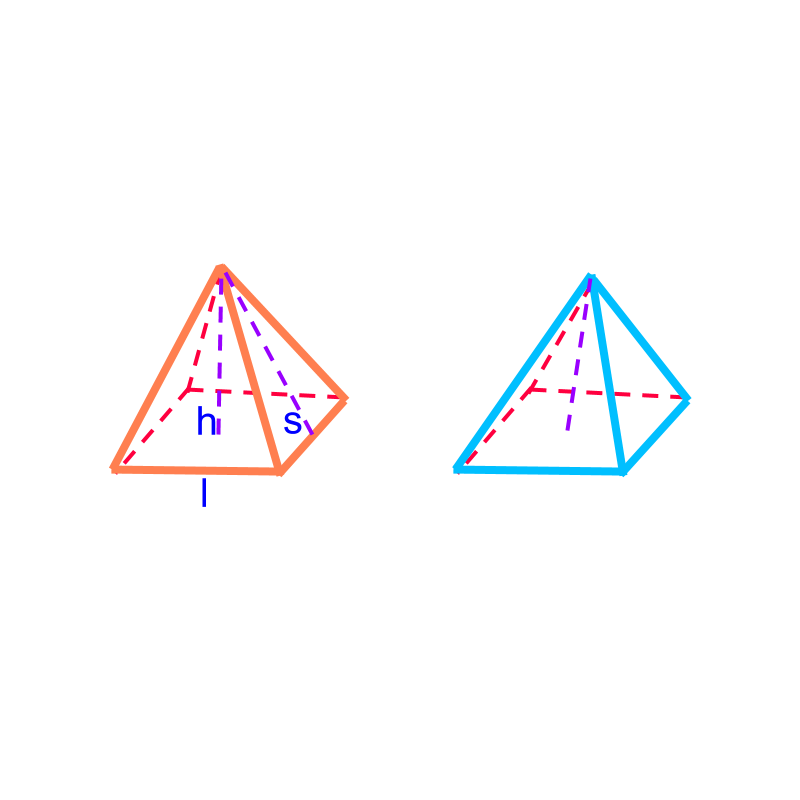We consider only right-pyramids with its axis at right angle to the base-surface. The oblique-pyramids have the angle between its axis and the base is not a right-angle. A right pyramid is shown in orange. And an oblique pyramid is shown in blue.

surface areaThe surface area of the square-pyramid of side $a$$a$ and height $h$$h$ is

$\phantom{\rule{1ex}{0ex}}\text{area of square}\phantom{\rule{1ex}{0ex}}$$\textrm{a r e a o f \square}$ $+4×\phantom{\rule{1ex}{0ex}}\text{area of triangles}$$+ 4 \times \textrm{a r e a o f \triangle s}$ or

$\phantom{\rule{1ex}{0ex}}\text{area of square}\phantom{\rule{1ex}{0ex}}$$\textrm{a r e a o f \square}$ $+\frac{1}{2}\phantom{\rule{1ex}{0ex}}\text{perimeter of base-surface}×\left(slantheight\right)$$+ \frac{1}{2} \textrm{p e r i m e t e r o f b a s e - s u r f a c e} \times \left(s l a n t h e i g h t\right)$

Note: A generic pyramid consists of

base-surface a 2D-polygon, (in this problem a square)

a set of triangular faces, (in this problem $5$$5$ triangles of base $a$$a$ and height $s$$s$).

$s$$s$ is the slant height computed as $\sqrt{{a}^{2}/4+{h}^{2}}$$\sqrt{{a}^{2} / 4 + {h}^{2}}$.A pyramid of height $h$$h$ is shown in orange. The triangular faces are shown in blue. Total surface area of the pyramid

$=$$=$ area of the base-surface $+$$+$ sum of the area of the triangular faces

volume of square pyramidThe volume of the square-pyramid of side $l$$l$ and height $h$$h$ is $\frac{1}{3}×\phantom{\rule{1ex}{0ex}}\text{area of base-surface}\phantom{\rule{1ex}{0ex}}$$\frac{1}{3} \times \textrm{a r e a o f b a s e - s u r f a c e}$ $×\phantom{\rule{1ex}{0ex}}\text{height}$$\times \textrm{h e i g h t}$

Note: As per the Cavalieri's principle in 3D, the pyramid is equivalently represented by the oblique-pyramid in blue.

The modified-pyramid fits into a cuboid. Each of the pyramids in blue, green, and purple, equal in volume, fit into the cuboid, and spans the entire cuboid. So, each fills exactly one-third of the cuboid as shown.

A square-pyramid of height $h$$h$ is shown in orange. As per the Cavalieri's principle in 3D, the pyramid is equivalently represented by the oblique-pyramid in blue.

The modified-pyramid fits into a cuboid. Each of the pyramids, shown on the lower half of the figure, in blue, green, and purple, are

•  equal in volume,

•  fit into the cuboid, and

•  spans the entire cuboid. So, each fills exactly one-third of the cuboid as shown.

Volume of the pyramid

$=$$=$ volume of the modified pyramid with identical cross sectional area along vertical axis

$=\frac{1}{3}×\phantom{\rule{1ex}{0ex}}\text{area of the base}\phantom{\rule{1ex}{0ex}}×\phantom{\rule{1ex}{0ex}}\text{height}$$= \frac{1}{3} \times \textrm{a r e a o f t h e b a s e} \times \textrm{h e i g h t}$

volume of any pyramid

The volume of the pentagonal-pyramid shown in the figure is $\frac{1}{3}×\phantom{\rule{1ex}{0ex}}\text{area of base-surface}\phantom{\rule{1ex}{0ex}}$$\frac{1}{3} \times \textrm{a r e a o f b a s e - s u r f a c e}$ $×\phantom{\rule{1ex}{0ex}}\text{height}$$\times \textrm{h e i g h t}$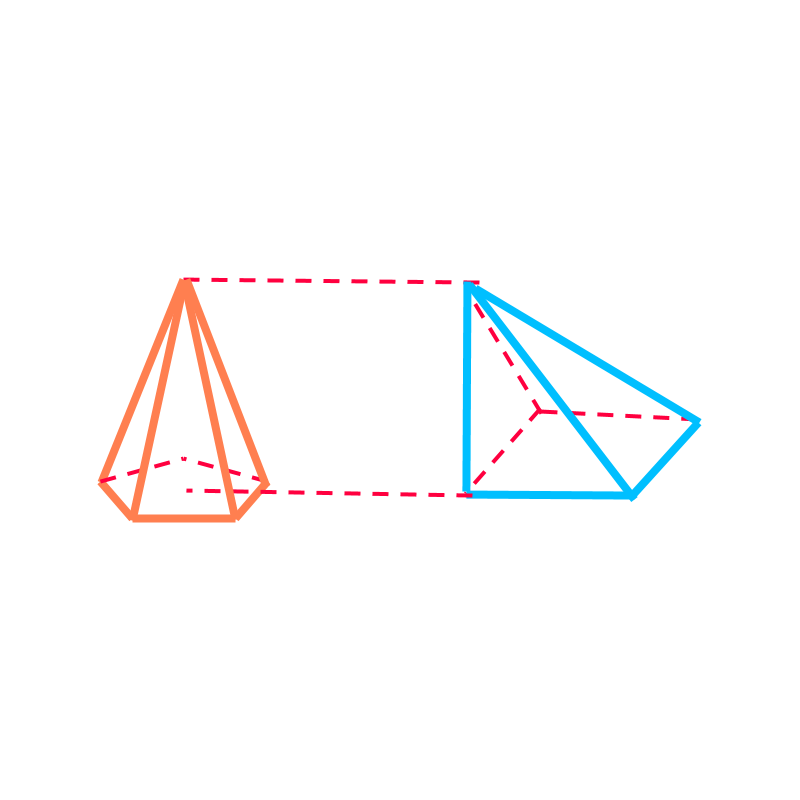A pentagonal-pyramid is shown in orange. As per the Cavalieri's principle in 3D, the pyramid is equivalently represented by the oblique-pyramid in blue.

The modified-pyramid has

•  the area at the bottom surface equal to the pentagonal pyramid

•  the height equal to the pentagonal pyramid.

•  the cross-sectional area at any point along vertical axis equals to the same of pentagonal pyramid

Volume of the pyramid

$=$$=$ volume of the modified pyramid with identical cross sectional area along vertical axis

$=\frac{1}{3}×\phantom{\rule{1ex}{0ex}}\text{area of the base}\phantom{\rule{1ex}{0ex}}×\phantom{\rule{1ex}{0ex}}\text{height}$$= \frac{1}{3} \times \textrm{a r e a o f t h e b a s e} \times \textrm{h e i g h t}$

What is the volume of a pyramid with base area $30c{m}^{2}$$30 c {m}^{2}$ and height $2$$2$ cm? Volume can be computed without specifying what type of pyramid is that. Volume $=\frac{1}{3}$$= \frac{1}{3}$ base-area $×$$\times$ height $=20c{m}^{3}$$= 20 c {m}^{3}$

summary

Surface Area and Volume of pyramid:Total surface area of the pyramid
$=$$=$ area of the base-surface$+$$+$ sum of the area of the side-facesVolume of the pyramid
$=\frac{1}{3}×\phantom{\rule{1ex}{0ex}}\text{area of the base-face}\phantom{\rule{1ex}{0ex}}×\phantom{\rule{1ex}{0ex}}\text{height}$$= \frac{1}{3} \times \textrm{a r e a o f t h e b a s e - f a c e} \times \textrm{h e i g h t}$

Outline